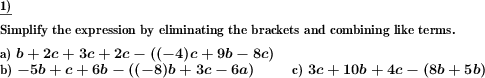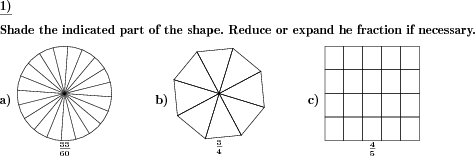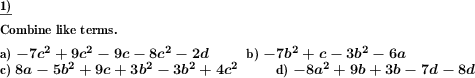Custom math worksheets at your fingertips# Details for problem "Combine like terms with negated brackets"

Quickname: 6625

Elementary School, Primary School, Junior High School, Middle School, High School.

## Summary

Combine like terms in a sum term and expand terms in negated brackets

## Example## Description

A term is made up of sums of multiples of powers of variables. Subterms in brackets may occur that have a negative sign. These bracketed terms have to be expanded properly. Like terms are terms that contain the same variables with the same exponents with different or same coefficients.

Like terms have to be combined to get the least number of terms possible. An upper limit may be specified to the greatest possible exponent so that terms with no exponents beyond one can be presented as a problem.

This simplifying algebraic expressions problem will strengthen skills at combining like terms and using brackets. It can be used in grades 5-7 to practice the skill of combining like terms with or without brackets in groups, or independent activity. Here are some results that can be achieved by mastering these types of problems.

1. The addition and subtraction of integers are revised.
2. Understanding of like terms by comparing variables and exponent is recalled.
3. Multiplication of signs is learned through this worksheet when dealing with brackets.
1. Apply the PEMDAS/BEDMAS/BODMAS rule to solve terms inside the brackets first.
2. Learn to use the commutative property to move all like terms together.
3. Practice adding the coefficients of like terms.
4. Learn to tidy up an expression or equation and make it more workable.

Personalize these worksheets to achieve differentiated learning outcomes.

• The number of problems from 1 to 10
• The number of variables from 2 to 6.
• The number of summands from 2, 3, 4.
• Choose exponents from 1 to 4.

The answer key provides a simplified expression with unique terms.

The above acronyms for the rules of precedence expand as follows for different countries:

PEMDAS
BEDMAS
BODMAS

Download free printable worksheets for this math problem here. The worksheet contains the problems only, the solution sheet includes the answers. Just click on the respective link.

•Worksheet 1Solution sheet with answers
•Worksheet 2Solution sheet with answers
•Worksheet 3Solution sheet with answers

If you can not see the solution sheets for download, they may be filtered out by an ad blocker that you may have installed. If this is the case, please allow ads for this page and reload the page. The solution sheets will then reappear.

• Do these sample worksheets do not really fit?
• Do you need more math worksheets, with a different level of difficulty?
• Would you like to combine different problems on a worksheet and adjust them to your needs?
• As a teacher, you can put together your own worksheets using the automatically generated math problems provided.
With a free initial credit, you can start creating your own math worksheets in a few minutes.

You can try it for free! Register here, to create custom worksheets now!

## Customization options for this problem

Parameter
Possible values
Number of problems
1, 2, 3, 4, 5
Number of variables
2, 3, 4, 5, 6
Number of summands
2, 3, 4
Greatest exponent
1, 2, 3, 4

## Similar problems

Remark
Description
With no bracketed terms
Combine like terms in a sum term
More complex with sum and product terms
Expand a term with sums and products with numbers and variables. Combine like terms to simplify the result.

## Other types of problems that appear on worksheets with this problem:

Relevance
Name
Description
Quickname
Example
****
Shade given fractions of a shape
An image of s subdivided shape is shown. Shade according to given fraction.****
Combine like terms
Combine like terms in a sum term****
Determine shapes with same shaded fraction
In a series of shapes find the two shapes that represent the same fraction.